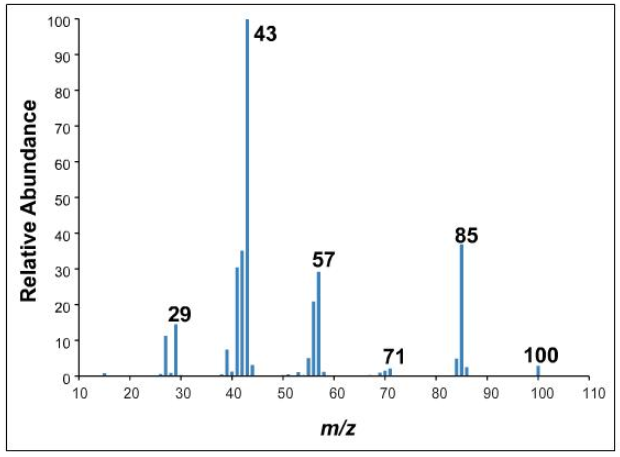# Problem: What is the molecular formula of the alkane?CnHx Replace the subscript letters with numbers. Formatting counts.

###### FREE Expert Solution

Use C-13 rule to determine the molecular formula of the compound

• By dividing the highest m/z value by 13, we can approximate the number of Cs in the molecule
• It was already mentioned that we have an alkane so we strictly have Cs and Hs on it.
95% (183 ratings)###### Problem Details

What is the molecular formula of the alkane?

CnH

Replace the subscript letters with numbers. Formatting counts.Frequently Asked Questions

What scientific concept do you need to know in order to solve this problem?

Our tutors have indicated that to solve this problem you will need to apply the Mass Spect: Fragmentation concept. You can view video lessons to learn Mass Spect: Fragmentation. Or if you need more Mass Spect: Fragmentation practice, you can also practice Mass Spect: Fragmentation practice problems.

What is the difficulty of this problem?

Our tutors rated the difficulty ofWhat is the molecular formula of the alkane?CnHx Replace the...as medium difficulty.

What professor is this problem relevant for?

Based on our data, we think this problem is relevant for Professor Mooring's class at GSU.